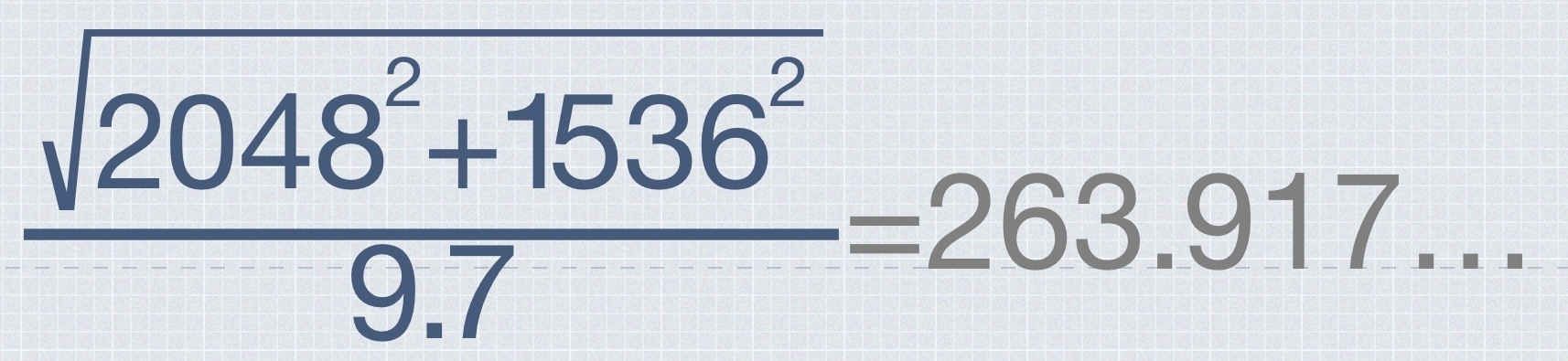# A Mutable Log

A blog by Devendra Tewari

Project maintained by tewarid Hosted on GitHub Pages — Theme by mattgraham

# PPI calculation

Have the screen resolution (width and height) in pixels and the diagonal length in inches, and want to calculate the PPI? Pretty simple really. The iPad 4 has a screen resolution of 2048 by 1536 pixels, and a diagonal length of 9.7 inches.

We can calculate its PPI asThe calculation above was performed using the wonderful MyScript Calculator, and the result captured by taking a screenshot.

The same formula can be written, expressed using $$\LaTeX$$, in a more generalized form as $$\sqrt{w^2 + h^2} \over L$$. Where, $$w$$ is the screen width in pixels, $$h$$ is the screen height in pixels, and $$L$$ is the diagonal length of the screen in inches.